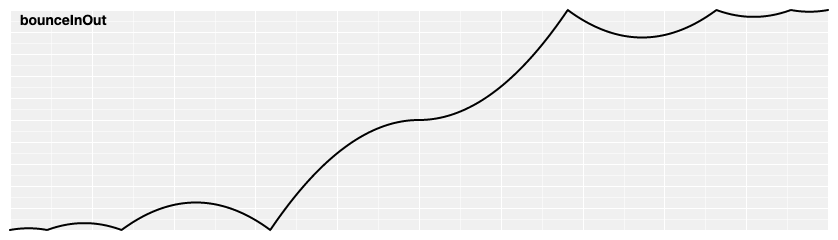# # d3-ease

Easing 是一种通过扭曲时间来控制动画中的表现形式的方法。通常被用来 slow-in, slow-out。通过对时间的缓动，animated transitions 会更平滑且运动过程也更合理。

## # Installing

`NPM` 安装: `npm install d3-ease`. 此外还可以下载 latest release. 可以直接从 d3js.orgstandalone library(单独的标准库) 或作为 D3 4.0 的一部分直接载入. 支持 `AMD`, `CommonJS`, 以及基础的标签引入形式。如果使用标签引入则会暴露 `d3` 全局变量:

``````<script src="https://d3js.org/d3-ease.v1.min.js"></script>
<script>

var ease = d3.easeCubic;

</script>
``````

## # API Reference

# ease(t)

``````var te = d3.easeCubic(t);
``````

``````// Before the animation starts, create your easing function.
var customElastic = d3.easeElastic.period(0.4);

// During the animation, apply the easing function.
var te = customElastic(t);
``````

# d3.easeLinear(t) <>

`Linear(线性)` 缓动。恒等函数，linear(t) 返回 t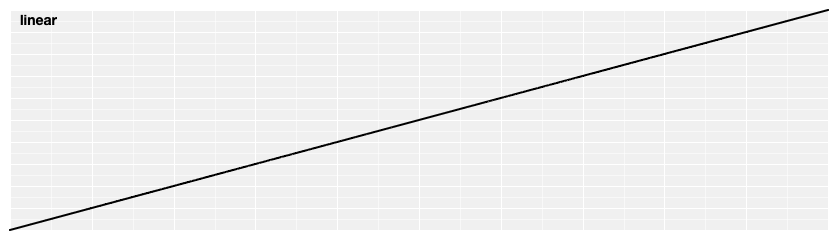# d3.easePolyIn(t) <>

`Polynomial(多项式)` 缓动; 将 t 进行 exponent 运算. 如果指数没有指定则默认为 `3`, 等价于 cubicIn.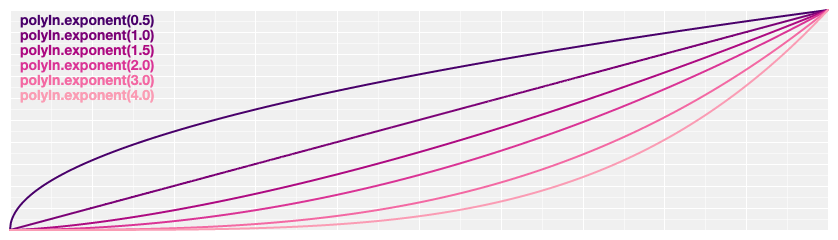# d3.easePolyOut(t) <>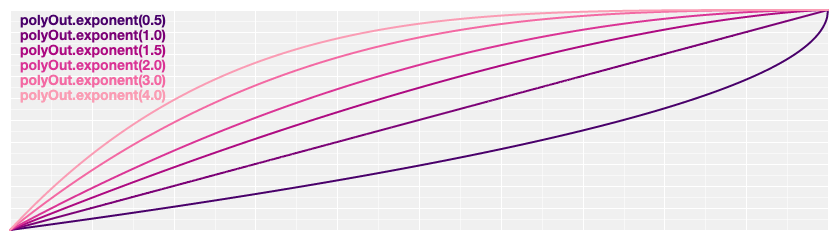# d3.easePoly(t) <>
# d3.easePolyInOut(t) <>

`Symmetric polynomial(对称多项式)` 缓动; 在 t 处于 [0, 0.5] 时使用 polyInt 处于 [0.5, 1] 时 使用 polyOut. 如果没有指定 exponent 则默认为 `3`. 等价于 cubic.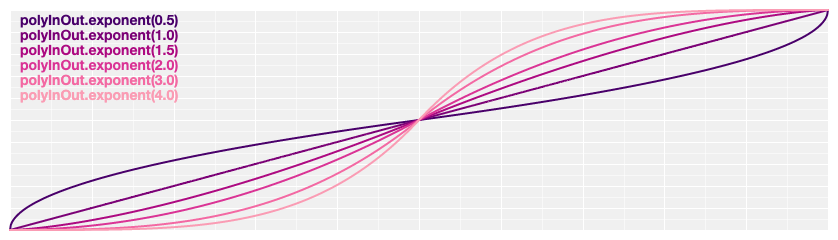# poly.exponent(e) <>

``````var linear = d3.easePoly.exponent(1),
cubic = d3.easePoly.exponent(3);
``````

`Quadratic(二次缓动)`; 等价于 polyIn.exponent(2).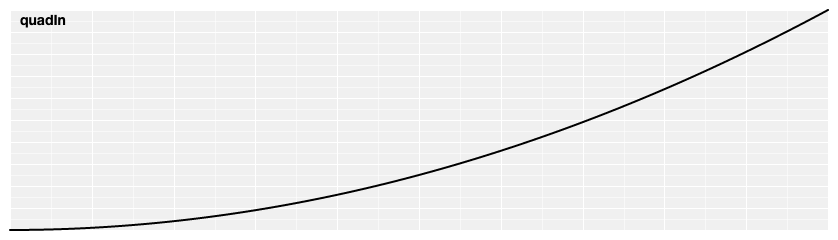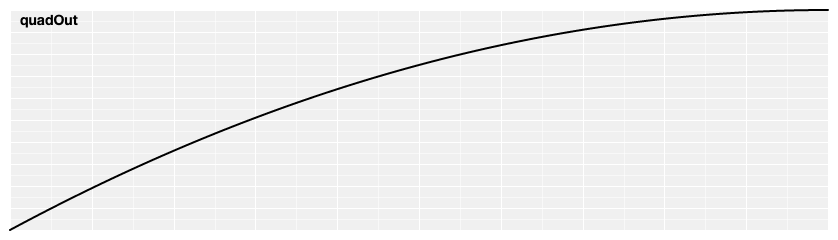`Symmetric quadratic(对称二次缓动)` ; t 处于[0, 0.5] 时使用 quadInt 处于 [0.5, 1] 时使用 quadOut. 也等价于 poly.exponent(2).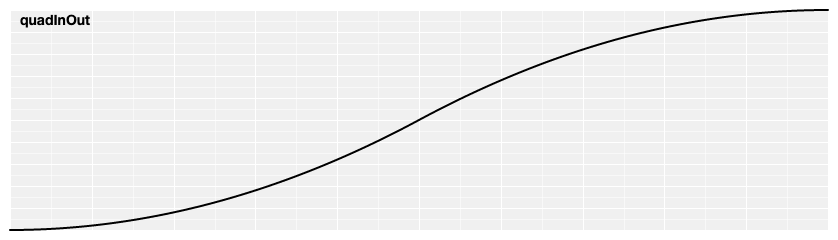# d3.easeCubicIn(t) <>

`Cubic(三次缓动)`；等价于 polyIn.exponent(3)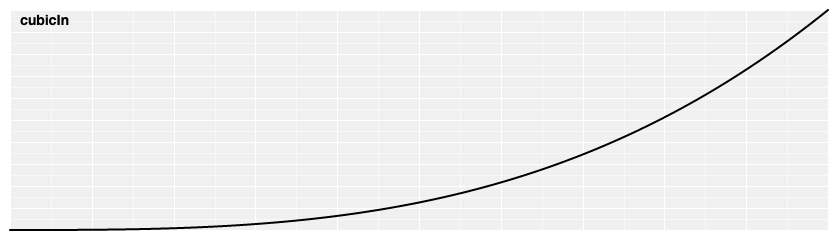# d3.easeCubicOut(t) <>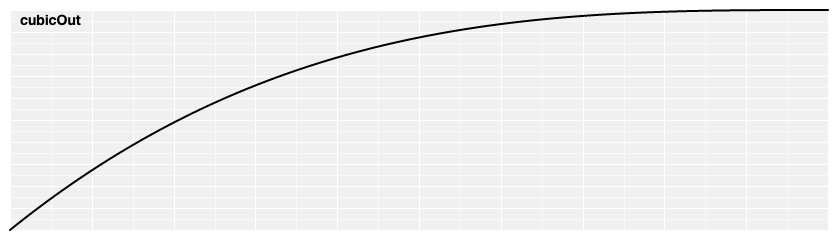# d3.easeCubic(t) <>
# d3.easeCubicInOut(t) <>

`Symmetric cubic(对称三次缓动)`; t 处于 [0, 0.5] 时使用 cubicInt 处于 [0.5, 1] 时使用 cubicOut。也等价于 poly.exponent(3).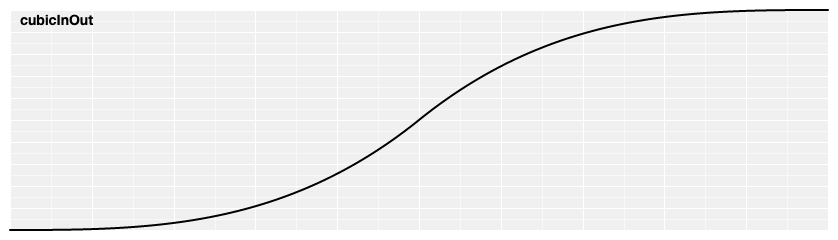# d3.easeSinIn(t) <>

`Sinusoidal(正弦缓动)`; 返回 `sin(*t*)`.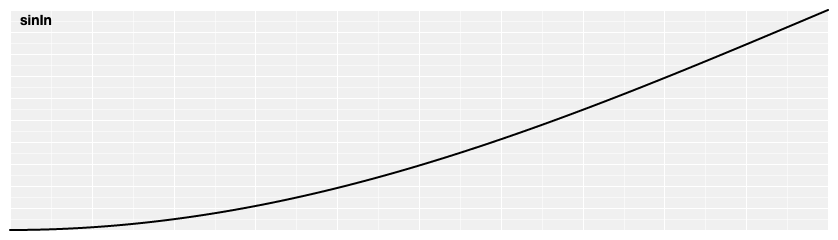# d3.easeSinOut(t) <>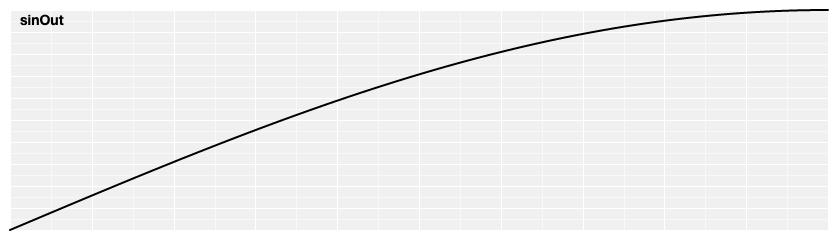# d3.easeSin(t) <>
# d3.easeSinInOut(t) <>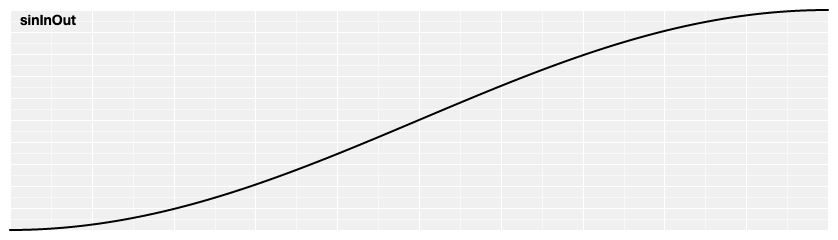# d3.easeExpIn(t) <>

`Exponential(指数)` 缓动; 映射为 2 的 10 * (t - 1) 次方.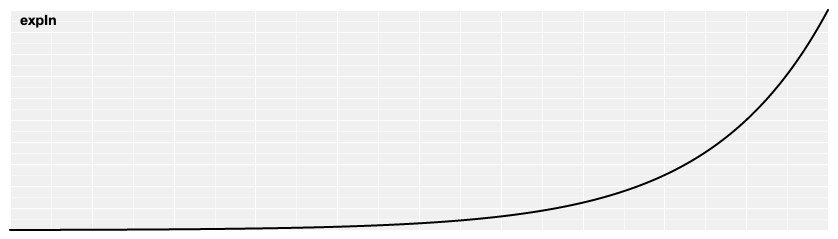# d3.easeExpOut(t) <>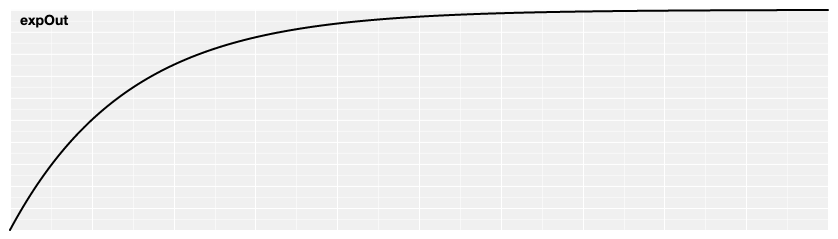# d3.easeExp(t) <>
# d3.easeExpInOut(t) <>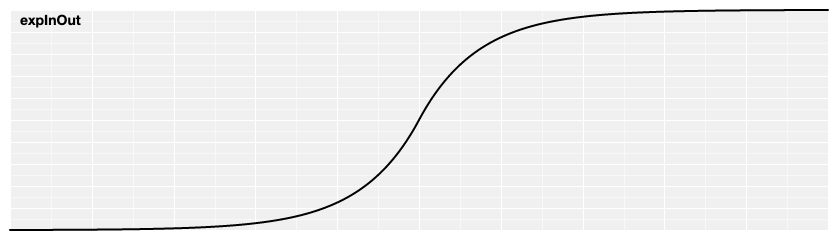# d3.easeCircleIn(t) <>

`Circular(环形缓动)`.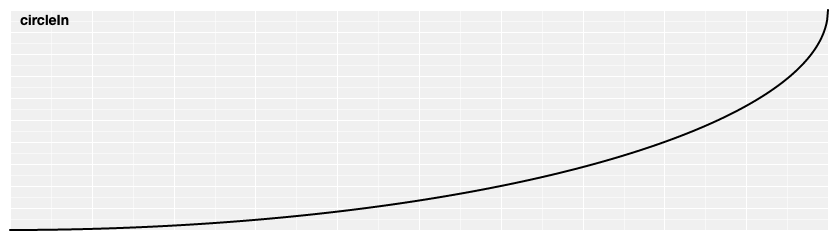# d3.easeCircleOut(t) <>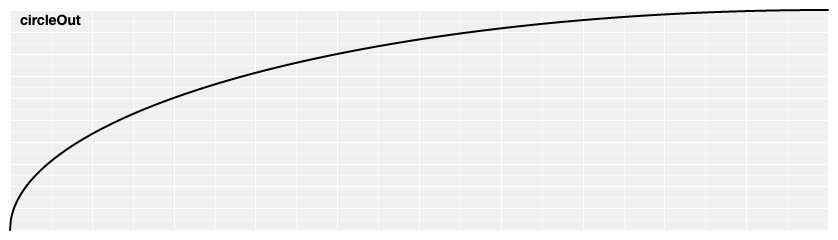# d3.easeCircle(t) <>
# d3.easeCircleInOut(t) <>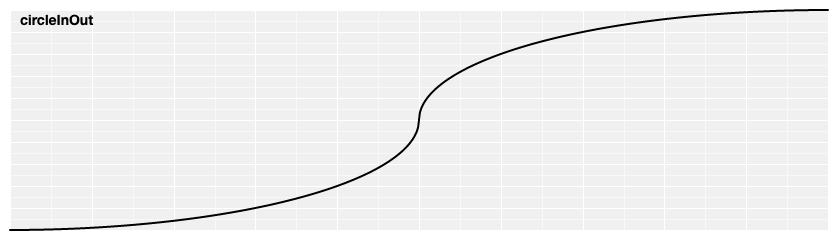# d3.easeElasticIn(t) <>

`Elastic(震荡缓动)`, 像一个震荡的橡皮筋. 震荡的 amplitude(振幅)period(周期) 是可以配置的; 如果没有指定则分别默认为 `1``0.3`.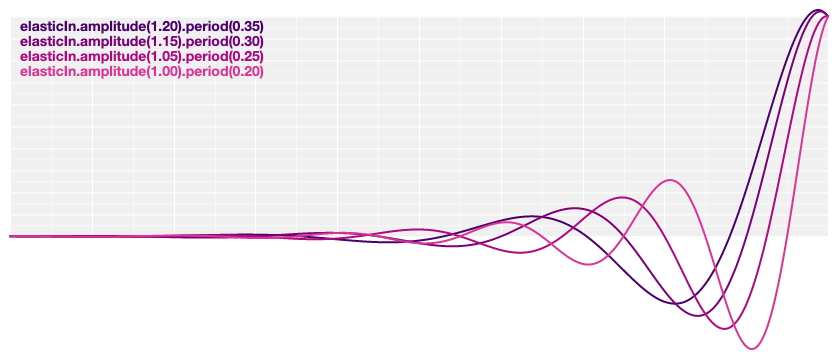# d3.easeElastic(t) <>
# d3.easeElasticOut(t) <>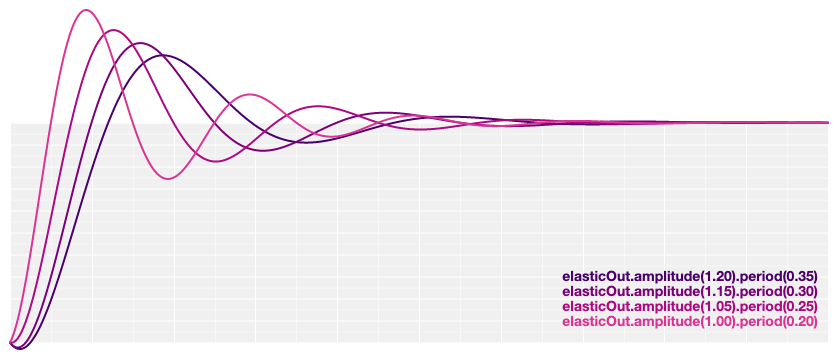# d3.easeElasticInOut(t) <>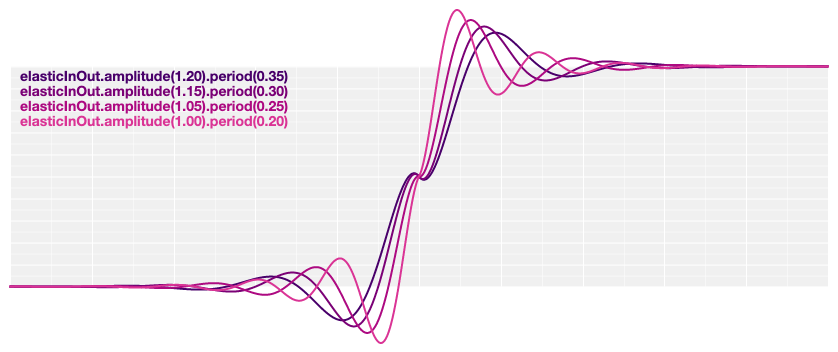# elastic.amplitude(a) <>

# elastic.period(p) <>

# d3.easeBackIn(t) <>

Anticipatory 缓动, 就像是一个舞者在跳跃之前先将自己的膝盖弯曲。 超调量 overshoot 是可配置的。如果没有指定的话默认为 `1.70158`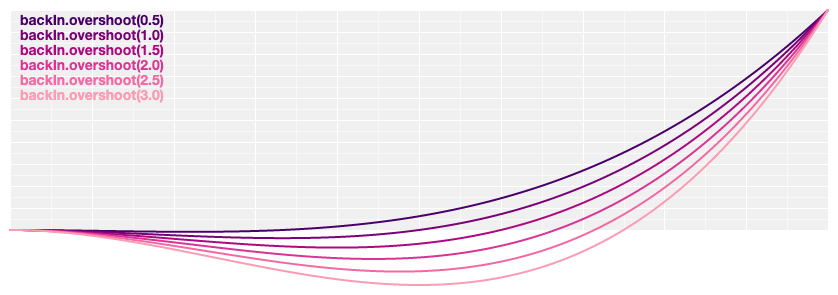# d3.easeBackOut(t) <>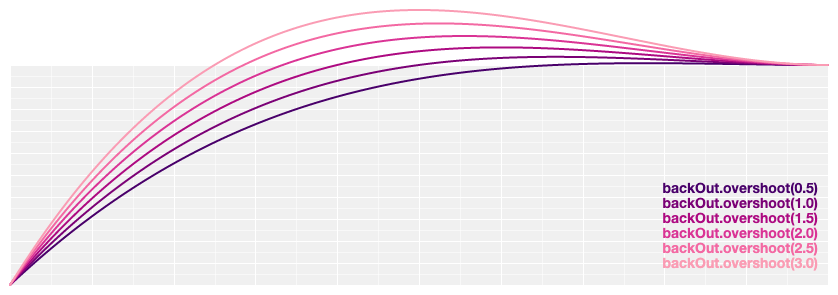# d3.easeBack(t) <>
# d3.easeBackInOut(t) <>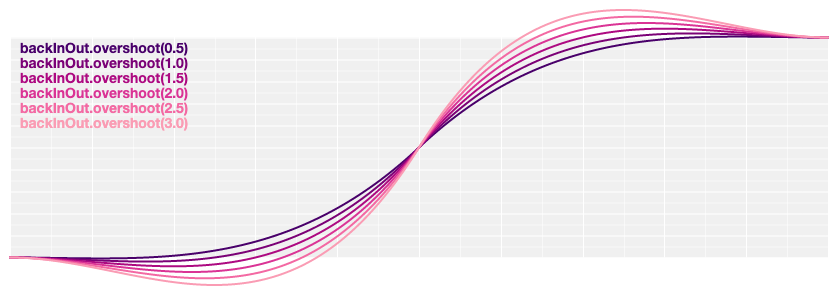# back.overshoot(s) <>

# d3.easeBounceIn(t) <>

`Bounce(弹跳缓动)`, 就像是一个橡皮球.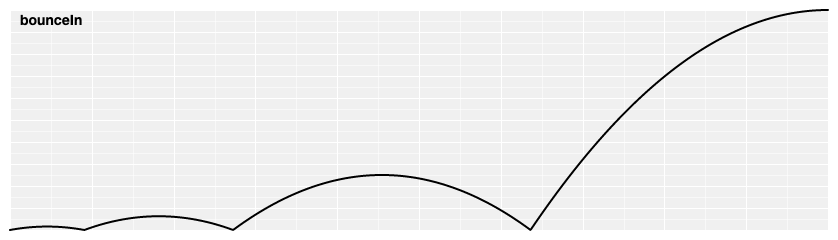# d3.easeBounce(t) <>
# d3.easeBounceOut(t) <>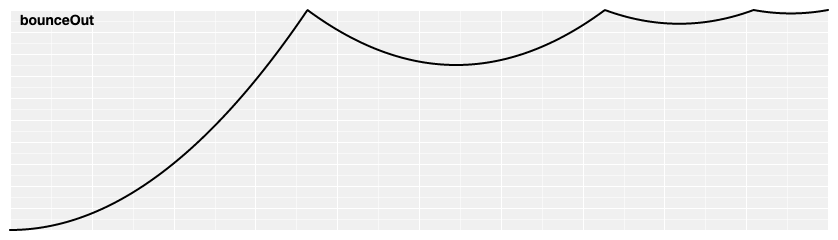# d3.easeBounceInOut(t) <>